## 散列表

散列表 ：根据给定的关键字来计算出关键字在表中的地址结构的数据结构。也就是说散列表建立了关键字存储地址之间的一种直接映射关系。

散列函数 ：一个把查找表中的关键字映射成该关键字对应的地址函数，记为 $Hash(key) = Addr$

散列函数可能会把两个或两个以上的不同关键字映射到同一地址，这种情况称为"冲突"，这些发生碰撞的不同关键字称为"同义词"

# 散列函数和冲突处理办法

• 散列函数的定义域必须包含全部需要存储的关键字，而值域的范围则依赖于散列表的大小或地址范围。
• 散列函数计算出来的地址应该能等概率、均匀地分布在整个空间，从而减少冲突的发生。
• 散列函数应尽量简单，能够在较短的时间内就计算出任一关键字对应的散列地址。

## 常用 Hash 函数的构造方法

### 1. 直接定址法

直接取关键字的某个线性函数值为散列地址，散列函数为 $H(key) = a * key + b$。式中，$a$ 和 $b$ 是常数。这种方法计算简单，并且不会产生冲突。

### 2. 除留余数法

假定散列表表长为 $m$，取一个不大于 $m$ 但最接近或等于 $m$ 的质数 $p$，利用以下公示把关键字转换成散列地址。散列函数为 $H(key) = key \% p$。除留余数法的关键是选好 $p$，使得每一个关键字通过该函数转换后等概率地映射到散列空间上的任一地址，从而尽可能减少冲突的可能性。

## 常用 Hash 函数的冲突处理办法：

### 1. 开放定址法

将产生冲突的 $Hash$ 地址作为自变量，通过某种冲突解决函数得到一个新的空闲的 $Hash$ 地址。

#### 1). 线性探测法：

发生冲突时，顺序查看表中下一个单元（当探测到表尾地址 $m-1$ 时，下一个探测地址是表首地址 $0$），直到找出一个空闲单元（当表填满时一定能找到一个空闲单元）或查遍全表。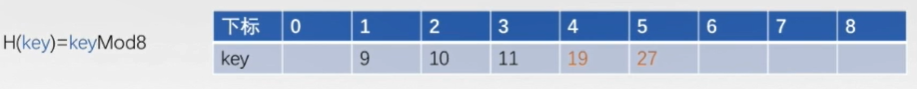当处理到 $19$ 时，通过哈希函数得到 $19$ 应该存放在 $3$的位置，但是 $3$ 的位置已经有元素，所以走到下一个位置，放到 $4$，的位置，同理 $27$，先看 $3$，然后看 $4$，最后放在 $5$ 的位置。

很显然，线性探测法会造成大量元素在相邻的散列地址上"聚集"起来，大大降低了查找效率。实现代码如下：

#include <bits/stdc++.h>
using namespace std;
int Hash;
int mod = 8;
int a;

inline void init(){
memset(Hash, -1, sizeof(Hash));
}

inline int Hash_key(int key){
return key % mod;
}

void Insert(int key){
int addr = Hash_key(key);
}
}

int Search(int key){
int addr = Hash_key(key);
int pre = addr;
while (Hash[addr] != key) {
if (Hash[addr] == -1 || Hash[addr] == pre) return -1;  // 返回了原点代表饶了一圈，此位置都为空了，说明值不存在，不然怎么也会放到这里
}
}

int main(){
init();
int n;
cin >> n;
for (int i = 1;i <= n;i++) {
cin >> a[i];
Insert(a[i]);
}
int x;
while (cin >> x) {
if (x == 0) break;
int pos = Search(x);
if (Hash[pos] == x)
cout << pos << "   " << Hash[pos] << endl;
else
cout << "no have" << endl;
}
system("pause");
return 0;
}
/*
5
9 10 11 19 27
*/


#### 2). 平方探测法

设发生冲突的地址为 $d$，平方探测法得到的新的地址序列为 $d + 12，d - 12，d + 22，d - 22......$ 平方探测法是一种较好的处理冲突的方法，可以避免出现"堆积"问题，它的缺点是不能探测散列表上的所有单元，但至少能探测到一半单元。#### 3). 再散列法

又称为双散列法。需要使用两个散列函数，当通过第一个散列函数的到 $H(key)$ 得到得地址发生冲突时，则利用第二个散列函数 $Hash2(key)$ 计算该关键字的地址增量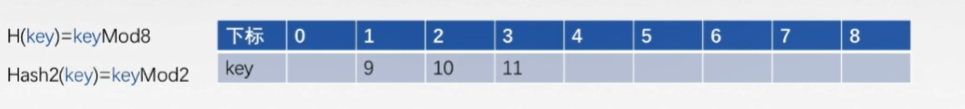$H_i = (Hash(key) + i*Hash2(key)) % m$，其中 $m$ 是散列表的长度， $i$ 是冲突次数，初值为 $0$。

比如现在在散列表中插入 $19$， $H(key) = 19 % 8 = 3$，产生第一次冲突 $i = 1$，带入 $H_i$ 计算，$h_i = (3 + 1*1) % 9 = 4$，于是 $19$ 插入到下标为 $4$，的位置。

### 2. 拉链法

对于不同的关键字可能会通过散列函数映射到同一地址，为了避免非同义词发生冲突，可以把所有同义词存储在一个线性链表中，这个线性链表由其散列地址唯一标识。拉链法适用经常插入和删除的情况。下面代码是一个拉链法的实现代码。

首先了解一个概念，装填因子，它与散列表的查找性能有关，散列表的装填因子一般定为 $\alpha$。

$$计算方法为 \alpha = \frac{表中记录数 n}{散列表长度 m}$$

散列表的平均查找长度依赖于散列表的装填因子 $\alpha$，而不直接依赖于 $n$ 或 $m$。$\alpha$越大，表示装填的记录越“满”，发生冲突的可能性就越大，反之发生冲突的可能性就越小。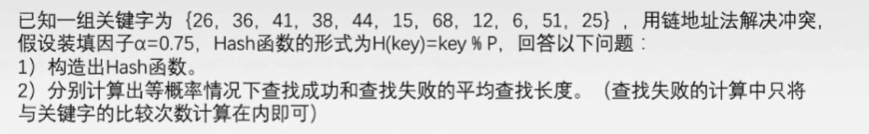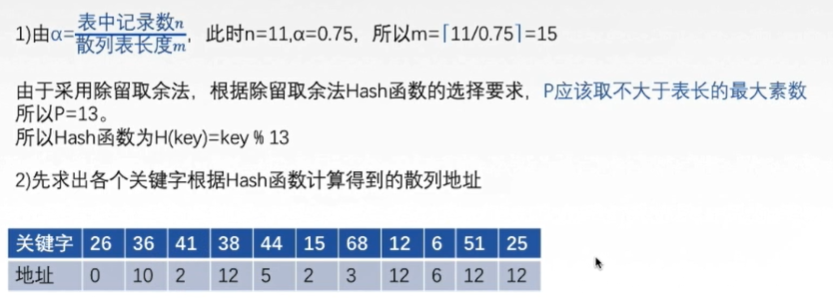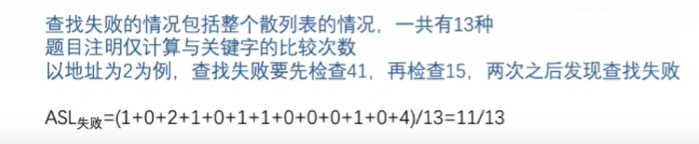#include <bits/stdc++.h>
using namespace std;
const int HASH_SIZE = 13;//hash表长度

struct Node{
int data;
struct Node *next;
};
static Node* HashTable[HASH_SIZE];//定义一个hash数组，该数组的每个元素是一个hash结点指针,并且由于是全局静态变量,默认初始化为NULL

unsigned int Hash_key(int key){
return key % HASH_SIZE;	//哈希函数
}

Node* LookUp(int key){
unsigned int HashValue = Hash_key(key);
Node* np = HashTable[HashValue];
//这里是链地址法解决的冲突,返回的是第一个链表结点
while(np){
//当两个数字相等时才能返回
if(np->data = key)
return np;
np = np->next;
}
return nullptr;
}

int Search(int key){
Node* np = LookUp(key);
if(np == nullptr)
return -1;
else
return np->data;
}

Node *malloc_Node(int key){
//在堆上为结点分配内存，并填充结点
Node *np = new Node;
if(np == nullptr)
return nullptr;

np->data = key;
np->next = nullptr;
return np;
}

int Insert(int key){
unsigned int HashValue;
HashValue = Hash_key(key);

Node* np = malloc_Node(key);
if(np == nullptr)
return 0;
//头插法，不管该hash位置有没有其他结点，直接插入结点
np->next = HashTable[HashValue];
HashTable[HashValue] = np;
return 1;
}

//显示hash表元素（不包括空）
void DisplayHashTable(){
Node* head = nullptr;
unsigned int HashValue;
for(int i = 0;i < HASH_SIZE;i++){
if(HashTable[i] != nullptr){
cout << "i = " << i << ":" << endl;
cout << head->data << " ";
}
cout << endl;
}
}
}

//清空hash表
void CleanHashTable(){
Node *head = nullptr, *pre = nullptr;
for(int i = 0;i < HASH_SIZE;i++){
if(HashTable[i] == nullptr)
continue;
}
}
}

int main(){
int a[] = {26, 36, 41, 38, 44, 15, 68, 12, 6, 51, 25};

for(int i=0; i < 11; ++i)
Insert(a[i]);

printf("we should see %d\n",Search(26));
Insert(41);//这里计算的hash是冲突的，为了测试冲突情况下的插入
DisplayHashTable();
CleanHashTable();

system("pause");
}


## 字符串哈希

### 自然溢出方法

unsigned long long HashTalbe[n]
HashTable[i] = HashTable[i−1] ∗ p + id(s[i])
//利用unsigned long long的范围自然溢出，相当于自动对2^64−1取模


### 单Hash方法

HashTable[i]=((HashTable[i−1]) ∗ p + id(s[i])) % mod；


其中 $p$ 和 $mod$ 均为质数，且有 $p < mod$。对于此种 $Hash$ 方法，将 $p$ 和 $mod$ 尽量取大即可，这种情况下，冲突的概率是很低的。对于到底多大 后面会放一张偷来的表。

如取p=13,mod=101，对字符串abcabc进行Hash
HashTable = 1
HashTable = (HashTable * 13 + 2) % 101 = 15
HashTable = (HashTable * 13 + 3) % 101 = 97



### 双Hash方法

hash1[i]=(hash1[i−1])∗p+idx(s[i]) % mod1
hash2[i]=(hash2[i−1])∗p+idx(s[i]) % mod2
//hash结果为<hash1[n],hash2[n]>
//这种Hash很安全。


### 获取子串的Hash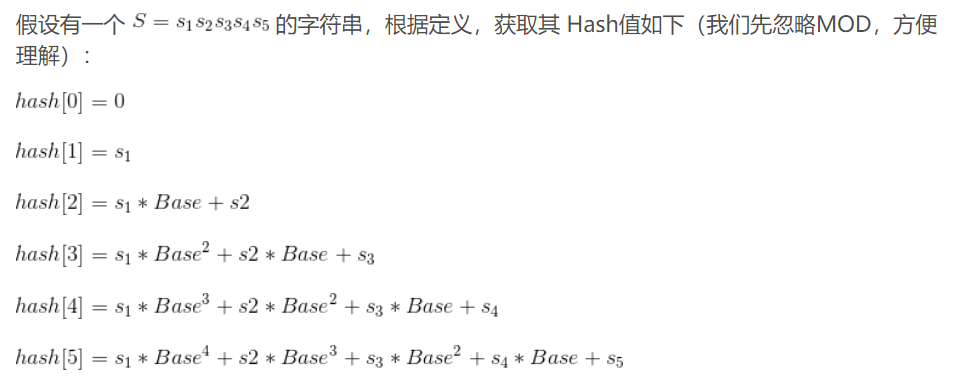$Hash = ((Hash[r] - hash[l - 1]* p ^ {r-l+1}) \% mod + mod) \% mod$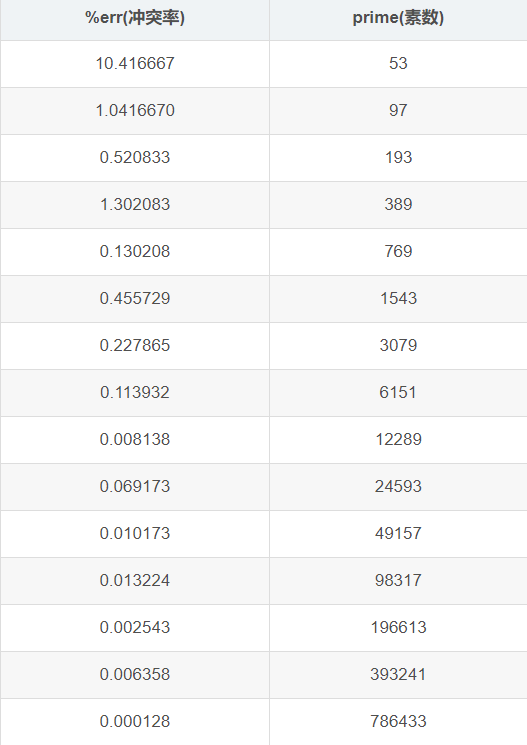class Solution {
public:
int distinctEchoSubstrings(string text) {
if(text.empty())
return 0;
text = "0" + text;
Hash.resize(text.size(), 0);
for(int i = 1;i < text.size();i++){
Hash[i] = (Hash[i-1]*p + (text[i] - 'a' + 1)) % mod;
}
for(int i = 1;i < text.size();i++){
for(int j = i + 1;j < text.size();j++){
if((j - i + 1) % 2 == 1)
continue;
int mid = (j + i) / 2;
long long l = ((Hash[mid] % mod - Hash[i - 1] % mod*QuickPow(p, mid - i + 1) % mod)% mod + mod) % mod;
long long r = ((Hash[j] % mod - Hash[mid + 1 - 1] % mod*QuickPow(p, j - (mid + 1) + 1) % mod)% mod + mod) % mod;
if(i == 4 && j == 413){
cout << "***********" << endl;
cout << l << " " << r << endl;
cout << vis[l] << endl;
cout << "***********" << endl;
}
if(l == r && l == 56090){
cout << "-----------" << endl;
cout << i << " " << j << endl;
cout << "-----------" << endl;
}
if(l == r && vis[l] == 0){
cout << i << " " << j << " " << l << endl;
vis[l] = 1;
ans++;
}
}
}
return ans;
}
private:
long long QuickPow(long long a, long long x){
long long ret = 1;
a %= mod;
while(x){
if(x & 1)
ret  = ret * a % mod;
a = a * a % mod;
x >>= 1;
}
return ret % mod;
}
private:
long long mod = 196613;
long long p = 24593;
vector<long long> Hash;
int ans = 0;
int vis = {0};
};